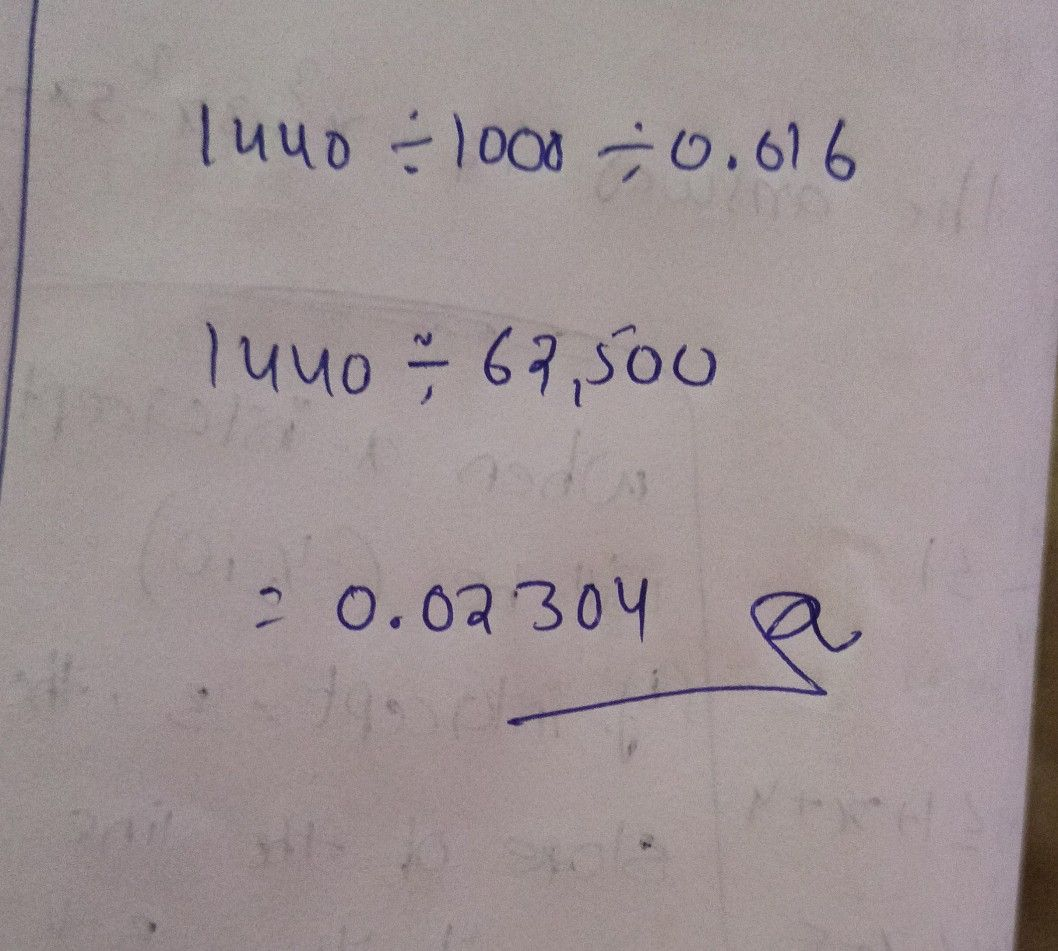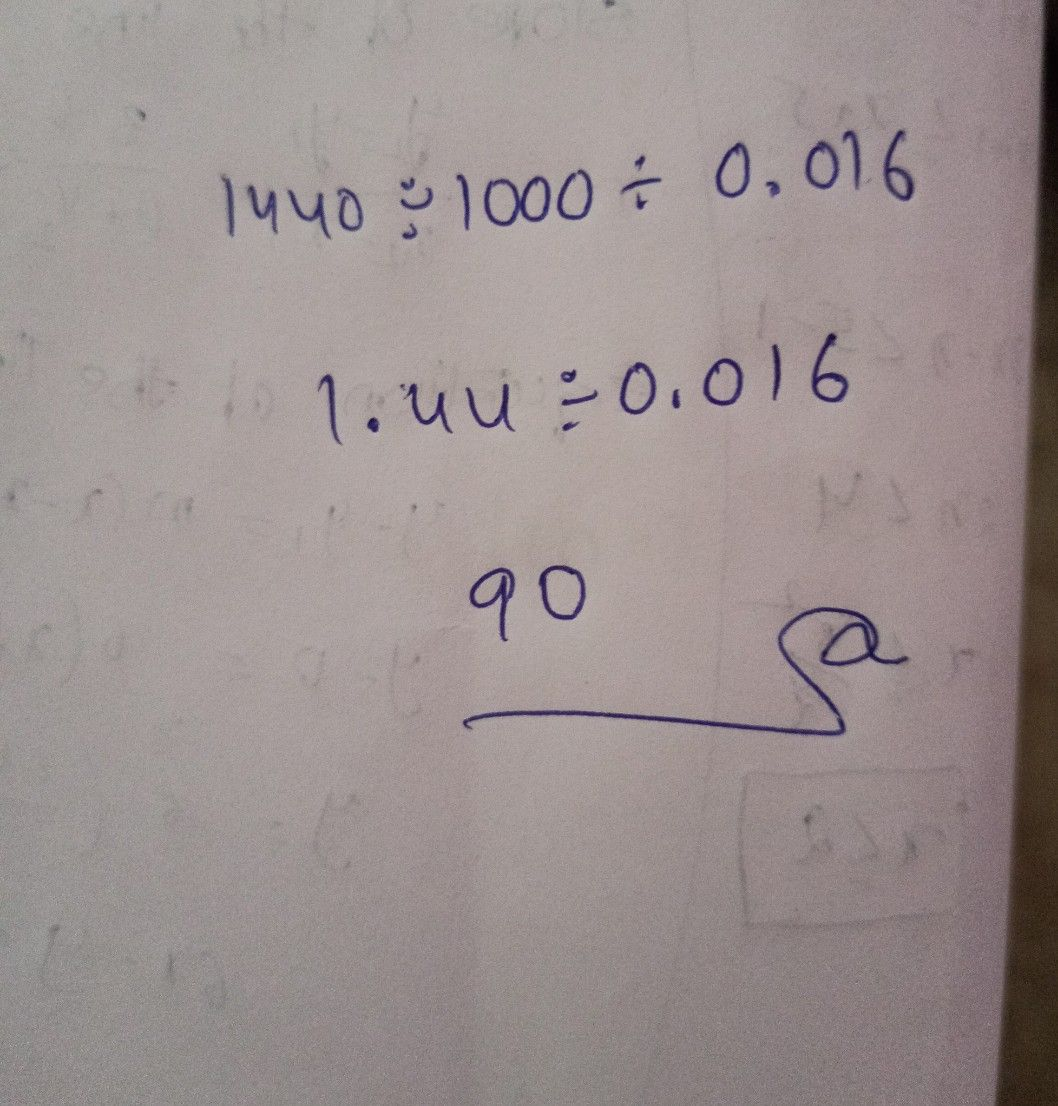Symbol
Problem$CL0$ = $0OC$ $+6.016$ $=$ ?How
10th-13th grade
Calculus
SolutionQanda teacher - shanviStudent
wrong answer
answer is 90Qanda teacher - shanvi
ok wait
I will checkok
give me satisfaction score
and give me some additional coins